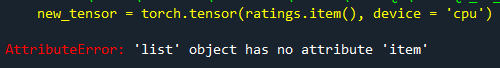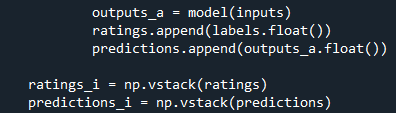# "can't convert cuda:0 device type tensor to numpy. Use Tensor.cpu() to copy the tensor to host memory first." and "list object has no attribute cpu"

Hello guys,

1. I have one of the common issues of type conversion “can’t convert cuda:0 device type tensor to numpy. Use Tensor.cpu() to copy the tensor to host memory first.”
So, I tried to solve like the answer comment " .cpu().numpy() ".
2. But unfortunately, another issue “list object has no attribute cpu.”
3. By trying to solve with “.cpu().detach().numpy()”, I got the error “list object has no attribute cpu”.
4. I also tried one of the related suggestions in this forum like “new_tensor = torch.tensor(old_tensor.item(), device = ‘cpu’)” but still have another issue “4. only one element tensors can be converted to Python scalars”.
5. The final attempt is trying using with .item() but still " AttributeError ‘list’ object has no attribute ‘item’ ".

So, if you have some idea or advice for me, please feel free to share.Hello,
According to me, `ratings` is probably a list of tensors.
Can you please confirm? Also, please brief what exactly you are trying to achieve.

Not sure if it helps your use case, but here’s a way to convert a list of tensors into a list of numpy arrays -

``````import torch
list_of_tensors = []
x = torch.tensor([1.0, 2, 3], device='cuda')
list_of_tensors.append(x)

x = torch.tensor([4.0, 5, 6], device='cuda')
list_of_tensors.append(x)

list_of_tensors
``````

out -

``````[tensor([1., 2., 3.], device='cuda:0'), tensor([4., 5., 6.], device='cuda:0')]
``````

Conversion -

``````list_of_arrays = [t.cpu().numpy() for t in list_of_tensors]
list_of_arrays
``````

out -

``````[array([1., 2., 3.], dtype=float32), array([4., 5., 6.], dtype=float32)]
``````

Thanks for your suggestions.

• In fact, I’ve also tested that way using .tensor function but got the value error “ValueError: only one element tensors can be converted to Python”.
• For the second way of using .cpu().numpy() in the loop got the same error " TypeError: can’t convert cuda:0 device type tensor to numpy. Use Tensor.cpu() to copy the tensor to host memory first. " as in my very first screenshot.Let me add the screenshot with that variable.

This error occured as you tried to put ratings (a list of tensors) in `torch.tensor`.

If you want to convert your list of tensors into a list of arrays, I’ve attached the code in my previous reply.

You can now use `np.vstack` on `list_of_arrays`.

As I asked earlier, please convey maybe by means of an example what exactly do you want to achieve.

1 Like

Thank you, Ms. Srishti. The append function is already used for the variable label (Tensor object) to the variable ratings (a list of Tensor). Here is the code that I am testing and got the above error.The types of variables:

Hi, please consider going through my previous reply again.

Use this:

``````ratings_arr = [t.cpu().numpy() for t in ratings]
ratings_i = np.vstack(ratings_arr)

predictions_arr = [t.cpu().numpy() for t in predictions]
predictions_i = np.vstack(predictions_arr)
``````

Let me know if you still face the error.